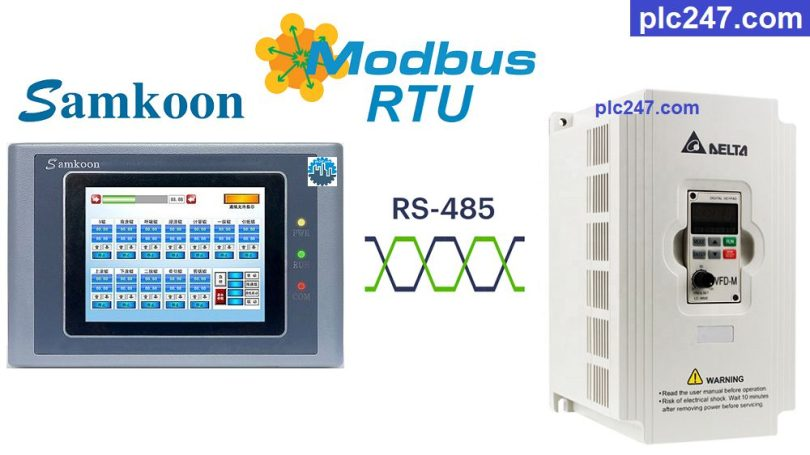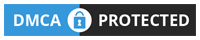# Samkoon HMI “Modbus RTU” Delta VFD-M TutorialWritten by

Hello friends. Delta VFD-M inverter is a type of inverter commonly used on the market (now discontinued), it fully supports the basic control connections of an inverter, including communication interface “Modbus-RTU” Communication Protocol.

Today plc247.com will guide you to use HMI Samkoon SK-070HS to control it simply and easily without connecting many wires.

#### Samkoon HMI & Delta VFD-M “Wiring Diagram”

RS485 communication connection diagram between Samkoon HMI and Delta VFD-M inverter (Delta VFD-M RS485 port is RJ11 port (desktop phone port).

#### Delta VFD-M Modbus Parameters Setting

Below are the Modbus-RTU communication parameters of Delta VFD-M inverter:

• P00 = “03” (Frequency Set by RS485)
• P01 = “04” ( Control by RS485)
• P88 = “01” (Slave Address)
• P89 = “01” (Baudrate 9600bps)
• P92 = “04” ( Mode 8/1/Even)
• P157 = “01” (Modbus-RTU Selection)
##### Delta VFD-M Modbus Registers Address

>>> VFD Control Address = 2000 (hex) = 8192 (dec)

+ Set Word 8192 = 18 (dec): Forward Run
+ Set Word 8192 = 34 (dec): Reverse Run
+ Set Word 8192 = 1 (dec): Stop

>>> Frequency Set Address = 2001 (hex) = 8194 (dec)

>>> Output Frequency Address = 2103 (hex) = 8451 (dec)

>>> Output Current Address = 2104 (hex) = 8452 (dec)

>>> Output Voltage Address = 2106 (hex) = 8454 (dec)

#### Samkoon HMI Modbus Configuration

We need to set the communication parameters of Delta VFD-M and HMI Samkoon the same:

### HMI Programming Example

+ Main Page Design

+ Setting Frequency

+ Forward Run

+ Reverse Run

+ Stop

+ Output Frequency

+ Output Current

+ Output Voltage

### Project Video Tutorial

======

###### Related Software and Documents